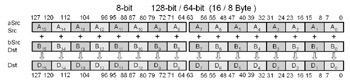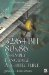Packed Addition and SubtractionAt this point the focus turns to the integer addition and subtraction of numbers in parallel. With the general-purpose instructions of a processor, normal calculations of addition and subtraction take place one at a time as scalar operations. These can instead be pipelined so that multiple integer calculations can occur simultaneously , but when performing large numbers of similar type calculations there is a bottleneck of processor calculation time. By using vector calculations, multiple like calculations can be performed simultaneously. The only trick here is to remember the key phrase "multiple like calculations."

If, for example, four pairs of 32-bit words are being calculated simultaneously such as in the following addition:

 47 53 38 87 + 23 + 74 + 39 + 16 70 127 77 103

or subtraction:

 47 53 38 87 23 74 39 16 24 21 1 71

the point is that the calculations all need to use the same operator. There is an exception, but this is too early to discuss it. There are workarounds, such as if only a couple of expressions need a calculated adjustment while others do not, then adding or subtracting a zero would keep their result neutral.

 47 53 38 87 + 23 + 0 + 39 + 0 70 53 77 87

Remember your algebraic law:

Algebraic Law :

 Additive Identity n + 0 = 0 + n = n

It is in essence a wasted calculation, but its use as a placeholder helps make SIMD instructions easier to use.

Algebraic Law :

 Additive Inverse a b = a + (b)

The other little item to remember is that subtraction is merely the addition of a value's additive inverse:

ab = a+(b)

PADDx destination , sourced (0n1 ) = a (0n1 ) + b (0n1) n={16, 8, 4, 2 }

Mnemonic

P

PII

K6

3D!

3Mx+

SSE

SSE2

A64

SSE3

E64T32/64-Bit 80x86 Assembly Language Architecture
ISBN: 1598220020
EAN: 2147483647
Year: 2003
Pages: 191

Similar book on Amazon